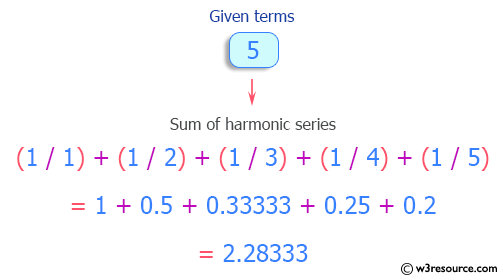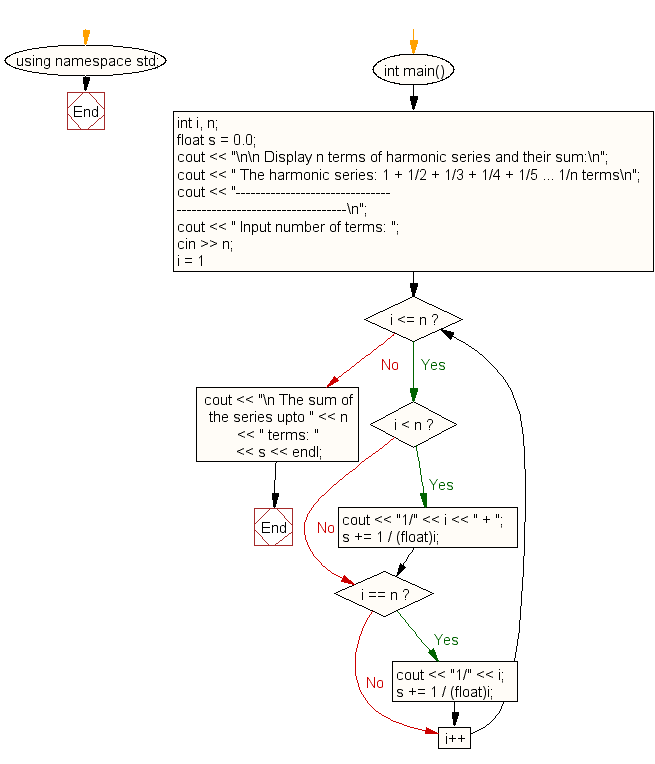﻿ C++ : Display the n terms of harmonic series and their sum

# C++ Exercises: Display the n terms of harmonic series and their sum

## C++ For Loop: Exercise-22 with Solution

Write a program in C++ to display the n terms of a harmonic series and their sum.
1 + 1/2 + 1/3 + 1/4 + 1/5 ... 1/n terms.

Pictorial Presentation:Sample Solution:-

C++ Code :

``````#include <iostream>
using namespace std;

int main()
{
int i, n;
float s = 0.0;
cout << "\n\n Display n terms of harmonic series and their sum:\n";
cout << " The harmonic series: 1 + 1/2 + 1/3 + 1/4 + 1/5 ... 1/n terms\n";
cout << "-----------------------------------------------------------------\n";
cout << " Input number of terms: ";
cin >> n;
for (i = 1; i <= n; i++)
{
if (i < n)
{
cout << "1/" << i << " + ";
s += 1 / (float)i;
}
if (i == n)
{
cout << "1/" << i;
s += 1 / (float)i;
}
}
cout << "\n The sum of the series upto " << n << " terms: " << s <<

endl;
}
``````

Sample Output:

``` Display n terms of harmonic series and their sum:
The harmonic series: 1 + 1/2 + 1/3 + 1/4 + 1/5 ... 1/n terms
-----------------------------------------------------------------
Input number of terms: 5
1/1 + 1/2 + 1/3 + 1/4 + 1/5
The sum of the series upto 5 terms: 2.28333
```

Flowchart:C++ Code Editor: